# Formulia

4.8

Formulia is an application focused on science students, mainly engineering. Its objective is to provide a collection of formulas from the different branches that exist in mathematics, physics and chemistry, as well as various other tools that will be helpful when performing certain calculations.

MATHS

● Algebra
● Geometry
● Flat and spherical trigonometry
● Differential calculus
● Integral calculus
● Multivariable calculus
● Probability and statistics
● Linear algebra
● Ordinary differential equations
● Fourier series and Laplace transforms
● Discrete mathematics
● Beta and Gamma functions
● Z Transform

PHYSICS

● Mechanics
● Fluid mechanics
● Waves
● Thermodynamics
● Electromagnetism
● Optical
● Modern Physics

CHEMISTRY

● Stoichiometry
● Solutions
● Thermochemistry
● Electrochemistry
● Gases
● Structure of the atom
● Organic chemistry

[NEW] Formulia Creator

Create, calculate and save your own formulas. This new functionality allows you to add custom calculators, with a huge variety of options, among the features it includes are:
● Classify your calculator by sections
● Add an unlimited number of variables, write its name and symbol, a description to know what it is about or its units of measurement with its conversion factor
● Program the formulas that you can calculate with each variable, thanks to the large number of operators that you can use
● Save the results of each calculation for later reference
● Share or import calculators with your classmates

TOOLS

● Universal constants
● Units of measure
● Unit conversions
● Tables of values ​​(densities, specific heats, etc.)
● Greek alphabet
● SI prefixes
● Mathematical symbology
● Scientific calculator
● Unit converter
● Matrix calculator

Periodic table: Check the most important information and properties of each chemical element such as:
● Electron configuration
● Atomic weight
● Oxidation states
● Number of electrons, protons and neutrons
● Density, melting and boiling point
● Heat of fusion, heat of vaporization and specific heat
● Thermal and electrical conductivity, and resistivity
● Electronegativity
● And other properties

The application is constantly growing and improving, any suggestion is welcome.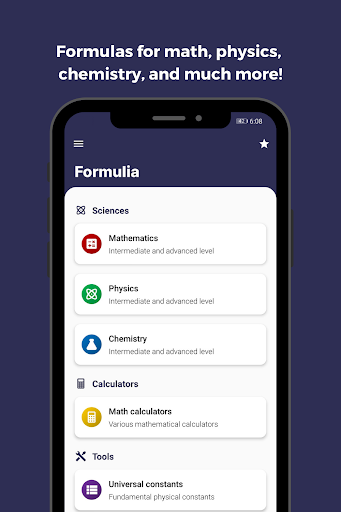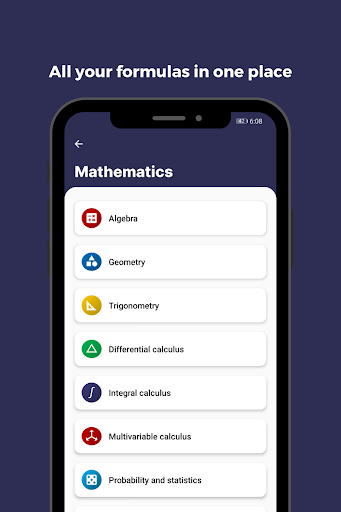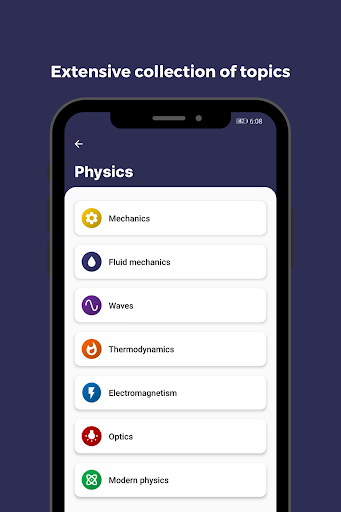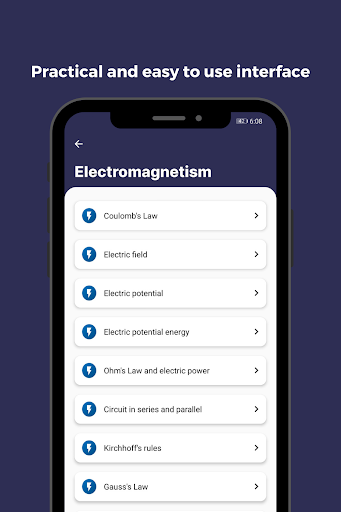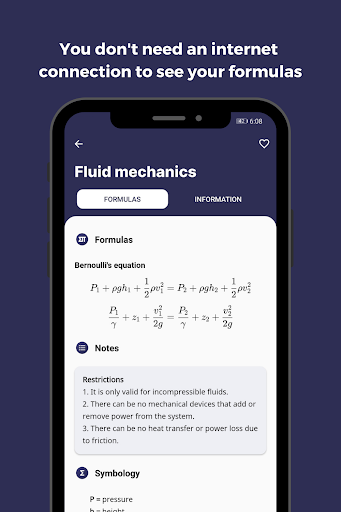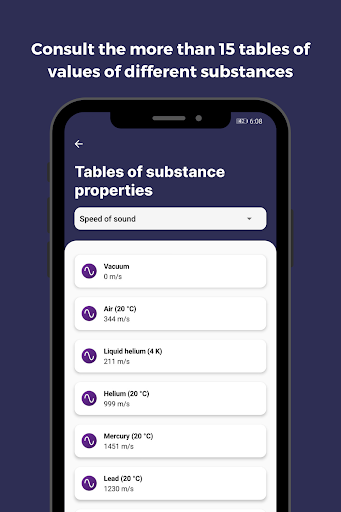##### Recent Updates - Version 6.2.1
• - UI improvements and dark mode support
- New units converter, with more than 40 magnitudes and great improvements